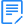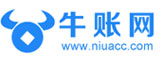# 完整版Excel快捷键汇总（电子版）

1、一对多查找

【例】根据产品查找相对应的所有供应商

A2 =B2&COUNTIF(B\$1:B2,B2)

B11=IFERROR(VLOOKUP(\$A11&COLUMN(A1),\$A:\$C,3,0),"")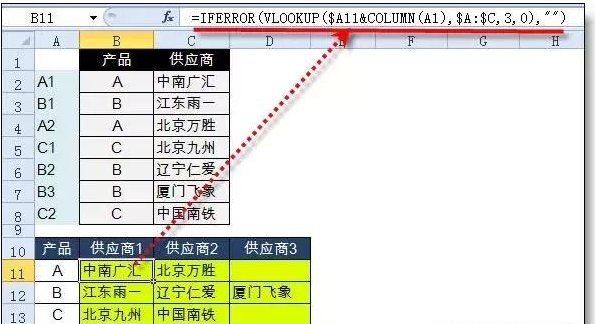2、查找销量最大的城市

{=INDEX(A:A,MAX((MAX(B3:B7)=B3:B7)*ROW(B3:B7)))}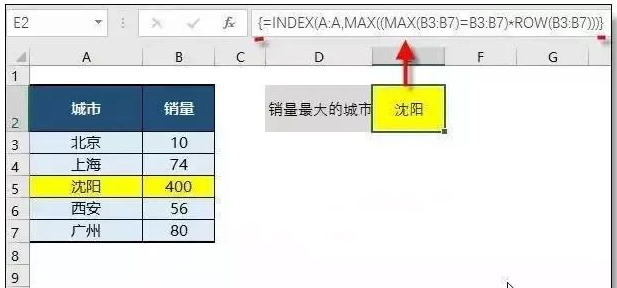3、最接近值查找

(数组公式按ctrl+shift+enter三键输入)

{=LOOKUP(1,0/(MIN(ABS(B3:B7-D4))=ABS(B3:B7-D4))*ROW(B3:B7),A3:A7)}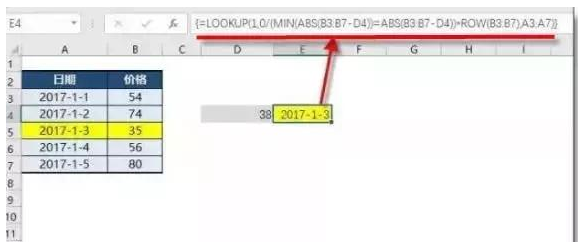4、跨多文件查找=VLOOKUP(A2,INDIRECT(LOOKUP(1,0/COUNTIF(INDIRECT("["&{"仓库1";"仓库2";"仓库3"}&".xlsx]sheet1!a:a"),A2),"["&{"仓库1";"仓库2";"仓库3"}&".xlsx]sheet1")&"!a:b"),2,0)。

vlookup函数的多文件查找，同样可以用iferror+vlookup的模式，公式虽然长，但容易理解且公式不容易出错。另外，如果excel文件有几十个或更多，就需要使用宏表函数用Files获取所有excel文件名称，然后应用到公式中，具体的公式就不再写了。

## 限时资料免费

•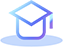重点汇总

•历年真题

•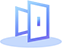章节练习

•备考攻略

•高分经验

•押题密卷

## 推荐课程